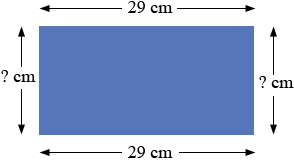SEARCH HOMEMath Central Quandaries & QueriesQuestion from Stacey, a student: If the length of rectangle is fixed at 29 cm, what widths will make the perimeter greater than 82 cm?Hi Stacey,

Draw a diagram!If the perimeter is 82 centimeters what is the lengths of the other two sides? Anything larger than that will yield a perimeter greater than 82 centimeters.

PennyMath Central is supported by the University of Regina and The Pacific Institute for the Mathematical Sciences.